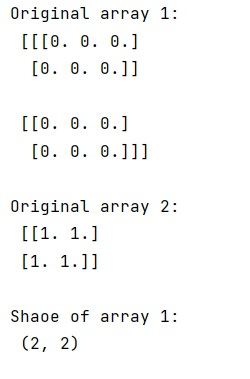# NumPy: Remove a dimension from np array

Learn, how to remove a dimension from NumPy array in Python?
Submitted by Pranit Sharma, on February 08, 2023

NumPy is an abbreviated form of Numerical Python. It is used for different types of scientific operations in python. Numpy is a vast library in python which is used for almost every kind of scientific or mathematical operation. It is itself an array which is a collection of various methods and functions for processing the arrays.

## Removing a dimension from NumPy array

As we know a picture contains pixels arranged an ndarray, suppose that we are working with some images some of which are 2-dimensional and others are 3-dimensional, we need to learn how to remove a dimension from an array so that we can efficiently work on these arrays.

Suppose that we are given two arrays of shape (100,100,3) and (100,100). The former means that we have 3 sets of things that have shape (100,100). Hence, to remove the last dimension, we just need to pick one of these dimensions using a fancy indexing technique.

Let us understand with the help of an example,

## Python code to remove a dimension from NumPy array

```# Import numpy
import numpy as np

# Creating two numpy arrays of different size
a1 = np.zeros((2,2,3))
a2 = np.ones((2,2))

# Display original arrays
print("Original array 1:\n",a1,"\n")
print("Original array 2:\n",a2,"\n")

# removing dimension of a1
res = a1[:,:,0]

# Display the result
print("Shaoe of array 1:\n",res.shape,"\n")
```

Output: